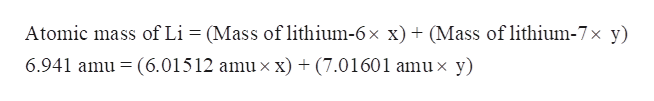# Lithium has only two naturally occurring isotopes. The mass of lithium-6 is 6.01512 amu and the mass of lithium-7 is 7.01601 amu.Use the atomic mass of lithium to calculate the relative abundances of the two isotopes.Express your answers using one decimal place. Enter your answers separated by a comma.

Question

Lithium has only two naturally occurring isotopes. The mass of lithium-6 is 6.01512 amu and the mass of lithium-7 is 7.01601 amu.

Use the atomic mass of lithium to calculate the relative abundances of the two isotopes.
check_circleExpert Solution
Step 1

Lithium has only two naturally occurring isotopes. The mass of lithium-6 is 6.01512 amu and the mass of lithium-7 is 7.01601 amu.

Using the atomic mass of lithium, we have to calculate the relative abundances of the two isotopes.

Step 2

If an element has two isotopes, then its average atomic mass is equal to the sum of mass of each isotope multiplied by its relative abundance.

Step 3

Average atomic mass of Lithium =6.941 amu

The mass of lithium-6 = 6.01512 amu

The mass of lithium-7 = 7...help_outlineImage TranscriptioncloseAtomic mass of Li (Mass of lithium-6x x) (Mass of lithium-7x y) 6.941 amu (6.01512 amu x x) + (7.01601 amu x y) fullscreen

### Want to see the full answer?

See Solution

#### Want to see this answer and more?

Solutions are written by subject experts who are available 24/7. Questions are typically answered within 1 hour*

See Solution
*Response times may vary by subject and question
Tagged in

### General Chemistry